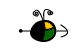Electrolysis

Electrolysis involves passing an electric current through either a molten salt or an ionic solution.  The ions are "forced" to undergo either oxidation (at the anode) or reduction (at the cathode).  Most electrolysis problems are really stoichiometry problems with the addition of an amount of electric current.  The quantities of substances produced or consumed by the electrolysis process is dependent upon the following:

• electric current measured in amperes or amps
• time measured in seconds
• the number of electrons required to produce or consume 1 mole of the substance
Amps, Time, Coulombs, Faradays, and Moles of Electrons

Three equations relate these quantities:

• amperes x time = Coulombs
• 96,485 coulombs = 1 Faraday
• 1 Faraday = 1 mole of electrons
The thought process for interconverting between amperes and moles of electrons is:

amps & time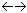CoulombsFaradaysmoles of electrons

Use of these equations are illustrated in the following sections.

Top

Calculating the Quantity of Substance Produced or Consumed

To determine the quantity of substance either produced or consumed during electrolysis given the time a known current flowed::

• Write the balanced half-reactions involved.
• Calculate the number of moles of electrons that were transferred.
• Calculate the number of moles of substance that was produced/consumed at the electrode.
• Convert the moles of substance to desired units of measure.
Example:   A 40.0 amp current flowed through molten iron(III) chloride for 10.0 hours (36,000 s).  Determine the mass of iron and the volume of chlorine gas (measured at 25oC and 1 atm) that is produced during this time.
• Write the half-reactions that take place at the anode and at the cathode.
anode (oxidation):  2 Cl-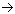Cl2(g) + 2 e-

cathode (reduction)  Fe3+ + 3 e-Fe(s)

• Calculate the number of moles of electrons.
•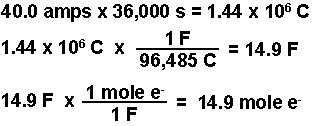• Calculate the moles of iron and of chlorine produced using the number of moles of electrons calculated and the stoichiometries from the balanced half-reactions.  According to the equations, three moles of electrons produce one mole of iron and 2 moles of electrons produce 1 mole of chlorine gas.
•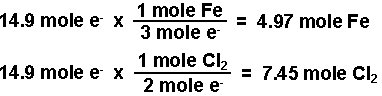• Calculate the mass of iron using the molar mass and calculate the volume of chlorine gas using the ideal gas law (PV = nRT).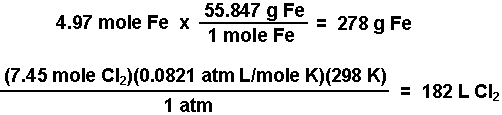Top

Calculating the Time Required

To determine the quantity of time required to produce a known quantity of a substance given the amount of current that flowed:

• Find the quantity of substance produced/consumed in moles.
• Write the balanced half-reaction involved.
• Calculate the number of moles of electrons required.
• Convert the moles of electrons into coulombs.
• Calculate the time required.
Example:  How long must a 20.0 amp current flow through a solution of ZnSO4 in order to produce 25.00 g of Zn metal.
• Convert the mass of Zn produced into moles using the molar mass of Zn.
•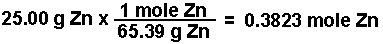• Write the half-reaction for the production of Zn at the cathode.
• Zn2+(aq) + 2 e-Zn(s)

• Calculate the moles of e- required to produce the moles of Zn using the stoichiometry of the balanced half-reaction.   According to the equation 2 moles of electrons will produce one mole of zinc.
•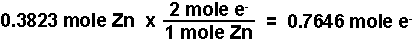• Convert the moles of electrons into coulombs of charge using Faraday's constant.
•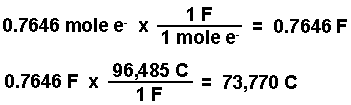• Calculate the time using the current and the coulombs of charge.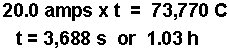Top

Calculating the Current Required

To determine the amount of current necessary to produce a known quantity of substance in a given amount of time:

• Find the quantity of substance produced/or consumed in moles.
• Write the equation for the half-reaction taking place.
• Calculate the number of moles of electrons required.
• Convert the moles of electrons into coulombs of charge.
• Calculate the current required.
Example:  What current is required to produce 400.0 L of hydrogen gas, measured at STP, from the electrolysis of water in 1 hour (3600 s)?
• Calculate the number of moles of H2. (Remember, at STP, 1 mole of any gas occupies 22.4 L.)
•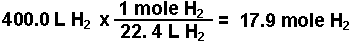• Write the equation for the half-reaction that takes place.
• Hydrogen is produced during the reduction of water at the cathode.  The equation for this half-reaction is:

4 e- + 4 H2O(l)2 H2(g) + 4 OH-(aq)

• Calculate the number of moles of electrons. According to the stoichiometry of the equation, 4 mole of e- are required to produce 2 moles of hydrogen gas, or 2 moles of e-'s for every one mole of hydrogen gas.
•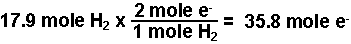• Convert the moles of electrons into coulombs of charge.
•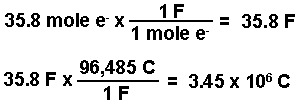• Calculate the current required.
•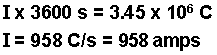Top